Statistical Physics MCQ Level – 2

# Statistical Physics MCQ Level – 2

Test Description

## 10 Questions MCQ Test Topic wise Tests for IIT JAM Physics | Statistical Physics MCQ Level – 2

Statistical Physics MCQ Level – 2 for IIT JAM 2023 is part of Topic wise Tests for IIT JAM Physics preparation. The Statistical Physics MCQ Level – 2 questions and answers have been prepared according to the IIT JAM exam syllabus.The Statistical Physics MCQ Level – 2 MCQs are made for IIT JAM 2023 Exam. Find important definitions, questions, notes, meanings, examples, exercises, MCQs and online tests for Statistical Physics MCQ Level – 2 below.
Solutions of Statistical Physics MCQ Level – 2 questions in English are available as part of our Topic wise Tests for IIT JAM Physics for IIT JAM & Statistical Physics MCQ Level – 2 solutions in Hindi for Topic wise Tests for IIT JAM Physics course. Download more important topics, notes, lectures and mock test series for IIT JAM Exam by signing up for free. Attempt Statistical Physics MCQ Level – 2 | 10 questions in 45 minutes | Mock test for IIT JAM preparation | Free important questions MCQ to study Topic wise Tests for IIT JAM Physics for IIT JAM Exam | Download free PDF with solutions
 1 Crore+ students have signed up on EduRev. Have you?
Statistical Physics MCQ Level – 2 - Question 1

### If Z be the partition function and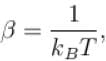then the average energy of the system is given by. Select one:

Detailed Solution for Statistical Physics MCQ Level – 2 - Question 1

Average energy of a system is defined as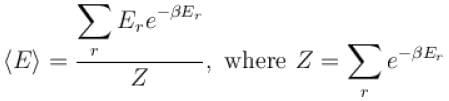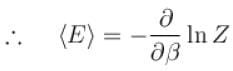The correct answer is: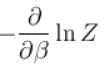Statistical Physics MCQ Level – 2 - Question 2

### For a Fermi gas of N particles in three dimension at T = 0K, the Fermi energy Ef is proportional to Select one:

Detailed Solution for Statistical Physics MCQ Level – 2 - Question 2

The Fermi energy is given by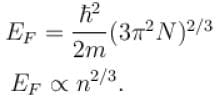Statistical Physics MCQ Level – 2 - Question 3

### Gibb’s paradox arises due to. Select one:

Detailed Solution for Statistical Physics MCQ Level – 2 - Question 3

In a semi-classical derivation of entropy that does not take into account the indistinguishability of particles, yields an expression for the entropy which is not extensive. This is called Gibb’s Paradox.
The correct answer is: Distinguishability of classical particles

Statistical Physics MCQ Level – 2 - Question 4

Which of the following relations between the particle number density n and temperature T must hold good for a gas consisting of non-interacting particles to be described by quantum statistics?
Select one:

Detailed Solution for Statistical Physics MCQ Level – 2 - Question 4

The particle density and temperature T are related as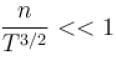The correct answer is: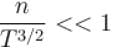Statistical Physics MCQ Level – 2 - Question 5

When an ideal quantum gas can be treated as an ideal classical gas?
Select one:

Detailed Solution for Statistical Physics MCQ Level – 2 - Question 5

Let us take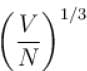where V is volume and N is number of particles.
Now, for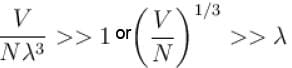i.e. when interparticle is much larger than the thermal de-Broglie wavelength, the gas will obey Maxwell Boltzmann Statistics.
The correct answer is: When de-Broglie wavelength is much smaller than the inter-particle distance

Statistical Physics MCQ Level – 2 - Question 6

What is the partition function of a monoatomic gas contained in value V, at temperature T and pressure p
Select one:

Detailed Solution for Statistical Physics MCQ Level – 2 - Question 6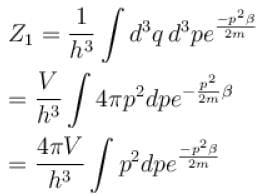Let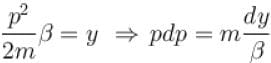∴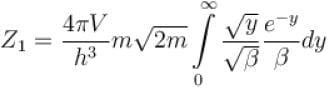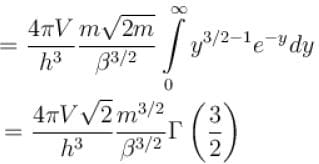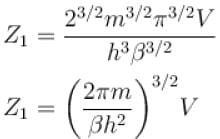∴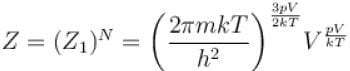The correct answer is: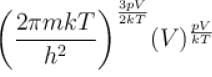Statistical Physics MCQ Level – 2 - Question 7

Consider a one level system having energy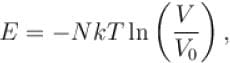where V0 is a constant and symbols have usual meanings, the partition function for the system is.
Select one:

Detailed Solution for Statistical Physics MCQ Level – 2 - Question 7

Partition function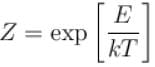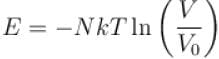⇒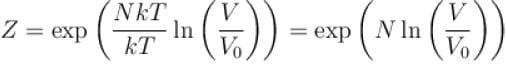The correct answer is: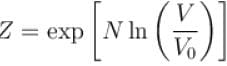Statistical Physics MCQ Level – 2 - Question 8

The partition function of a single gas molecule is Zα. The partition function of N such non-interacting gas molecules is then given by
Select one:

Detailed Solution for Statistical Physics MCQ Level – 2 - Question 8

Partition function of single gas molecule = Zα
Since N particles are non-interacting, the partition function =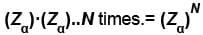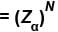The correct answer is: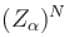Statistical Physics MCQ Level – 2 - Question 9

The classical partition function Z gives the
Select one:

Detailed Solution for Statistical Physics MCQ Level – 2 - Question 9

Classical partition function Z gives nothing but sum of all the possible states. In discrete systems, it gives basically the sum over all the microstates and in continuous systems it is represented in terms of integrating.
The correct answer is: Sum of states of the system

Statistical Physics MCQ Level – 2 - Question 10

For a single particle of mass m enclosed in a volume V. the number of accessible microstate in energy range E to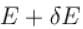is given by the (the phase space is divided by the rule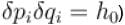Select one:

Detailed Solution for Statistical Physics MCQ Level – 2 - Question 10

The phase space volume is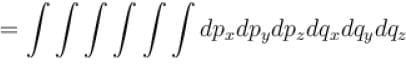where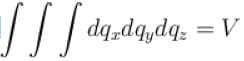and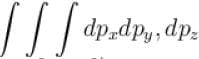is limited by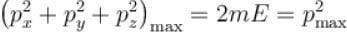Hence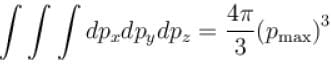∴ Volume of phase space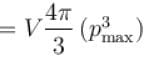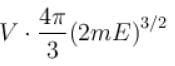Number of phase space =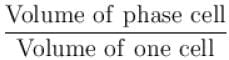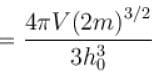The number of microstates in the energy range E to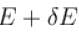is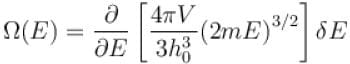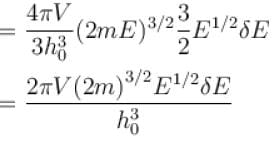The correct answer is: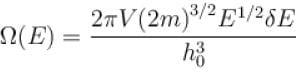## Topic wise Tests for IIT JAM Physics

217 tests
 Use Code STAYHOME200 and get INR 200 additional OFF Use Coupon Code
Information about Statistical Physics MCQ Level – 2 Page
In this test you can find the Exam questions for Statistical Physics MCQ Level – 2 solved & explained in the simplest way possible. Besides giving Questions and answers for Statistical Physics MCQ Level – 2, EduRev gives you an ample number of Online tests for practice

217 tests